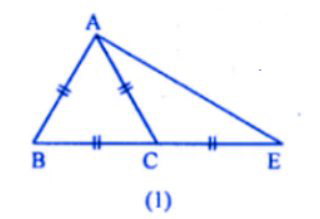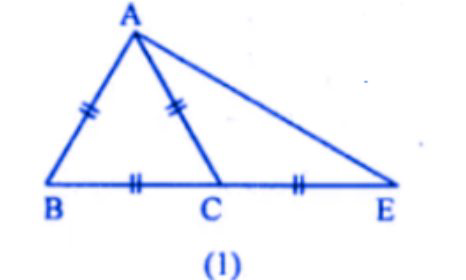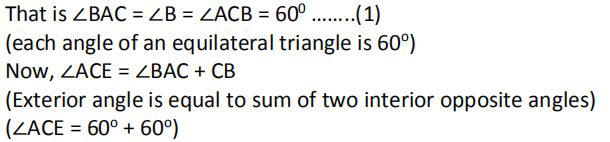# ML Aggarwal Solutions Class 9 Mathematics Solutions for Triangles Exercise 10.3 in Chapter 10 - Triangles

(a) In the figure (1) given below, ABC is an equilateral triangle. Base BC is produced

to E, such that BC’= CE. Calculate ∠ACE and ∠AEC.

(b) In the figure (2) given below, prove that ∠ BAD: ∠ ADB = 3: 1.

(c) In the figure (3) given below, AB || CD. Find the values of x, y, and ∠.(a) in the following figure

Given. ABC is an equilateral triangle BC = CE

To find. ∠ACE and ∠AECAs given that ABC is an equilateral triangle,Video transcript
"hey guys welcome to lido q a video i am vinit your leader tutor bringing you this question on your screen in the figure one given below abc is an equilateral triangle bc is produced to e such that bc is equal to c calculate ace and angle aec so angle ac is this an angle aec is this right now since triangle abc is equilateral angle abc is equal to angle acb is equal to angle cab is equal to 60 degree right because equilateral triangle great now angle angle ace is equal to angle abc plus angle ca b because exterior angle property is equal to 60 degree plus 60 degrees is equal to 120 degree this is our first answer now this is 120 degrees these two are bases right since triangle ace is isosceles angle aec equal to angle cae now angle aec plus angle cae equal to 180 degree minus 120 degrees again angle sum property right therefore we can say twice angle aec is equal to 60 degrees this implies angle aec is equal to 30 degrees isn't that easy guys right so if we still have a doubt please leave a comment below we'll try to get back to you do like the video and subscribe to our channel i will see you in our next video until then bye guys keep practicing"
Related Questions

Lido

Courses

Teachers

Book a Demo with us

Syllabus

Maths
CBSE
Maths
ICSE
Science
CBSE

Science
ICSE
English
CBSE
English
ICSE
Coding

Terms & Policies

Selina Question Bank

Maths
Physics
Biology

Allied Question Bank

Chemistry
Connect with us on social media!